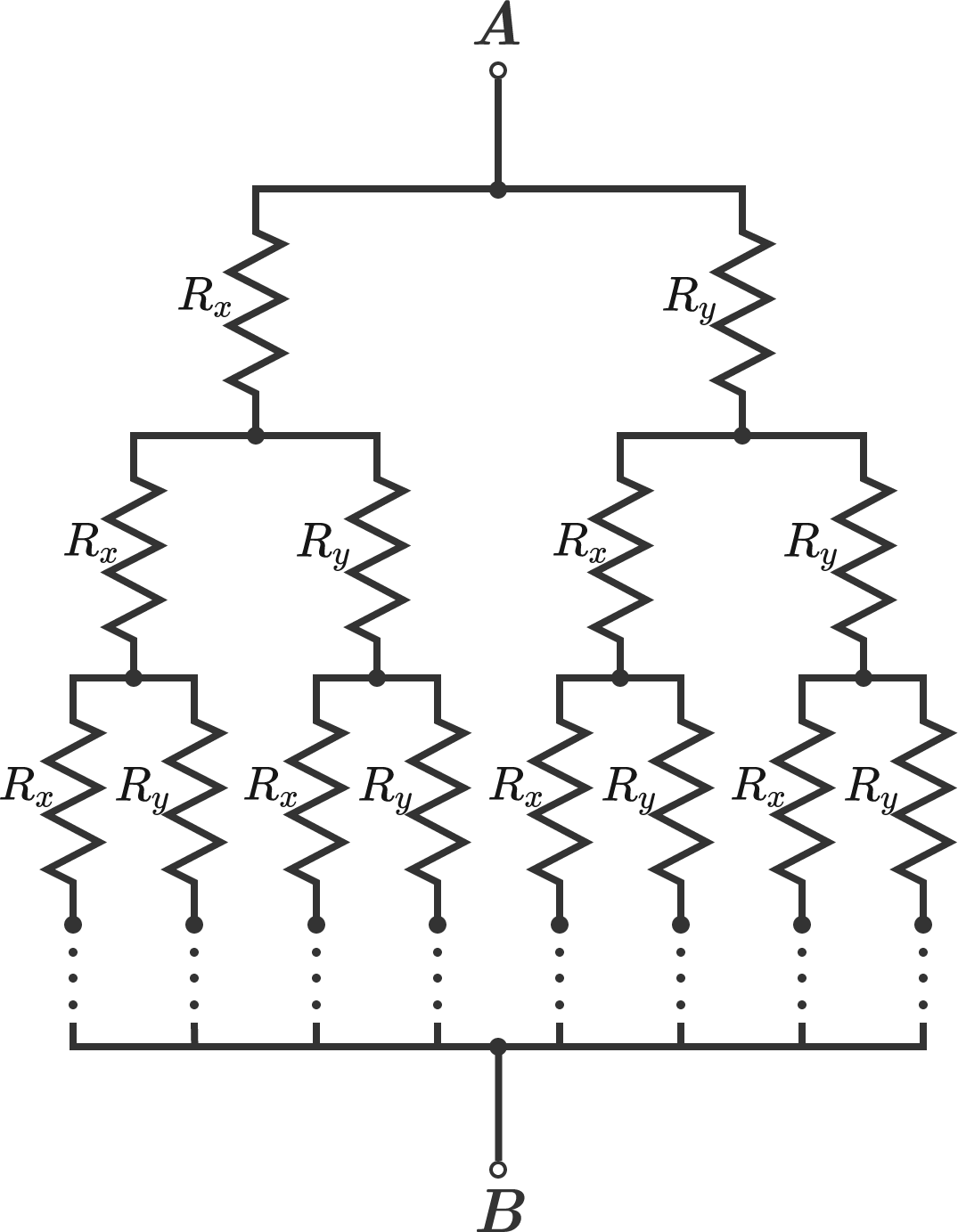# Resistors and meansThis resistive network forms an infinite binary tree--every branch splits into two new branches, where the new branch that goes to the left has a resistance of $R_x$, and the one that goes to the right has a resistance of $R_y$.

Suppose that after going down $n$ levels, all of the branches are connected to a single node, $B$. As $n$ approaches infinity, what will be the equivalent resistance between nodes $A$ and $B?$

×

Problem Loading...

Note Loading...

Set Loading...